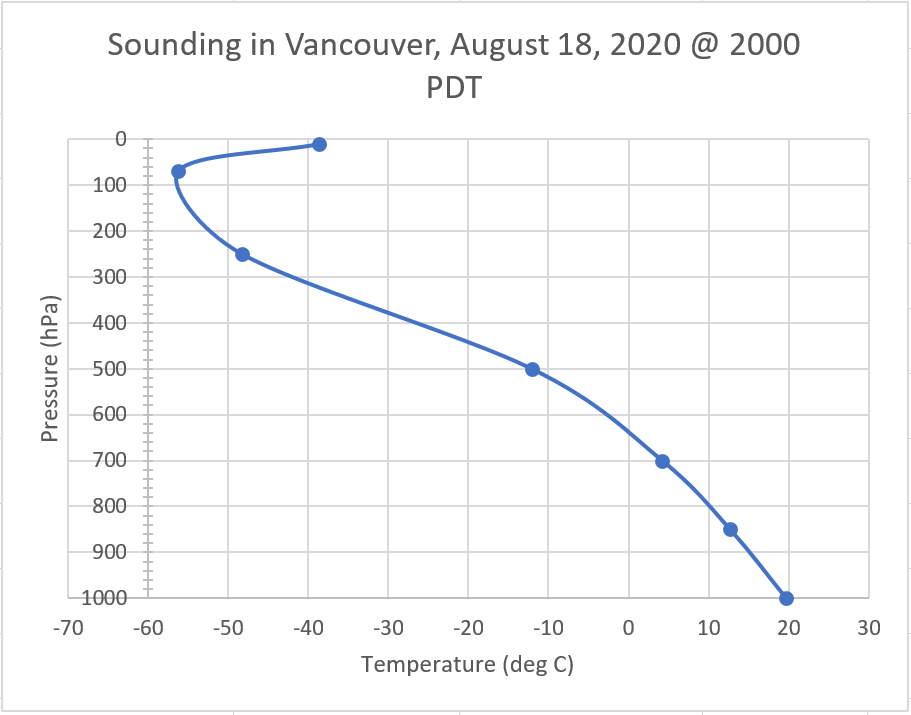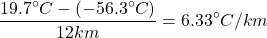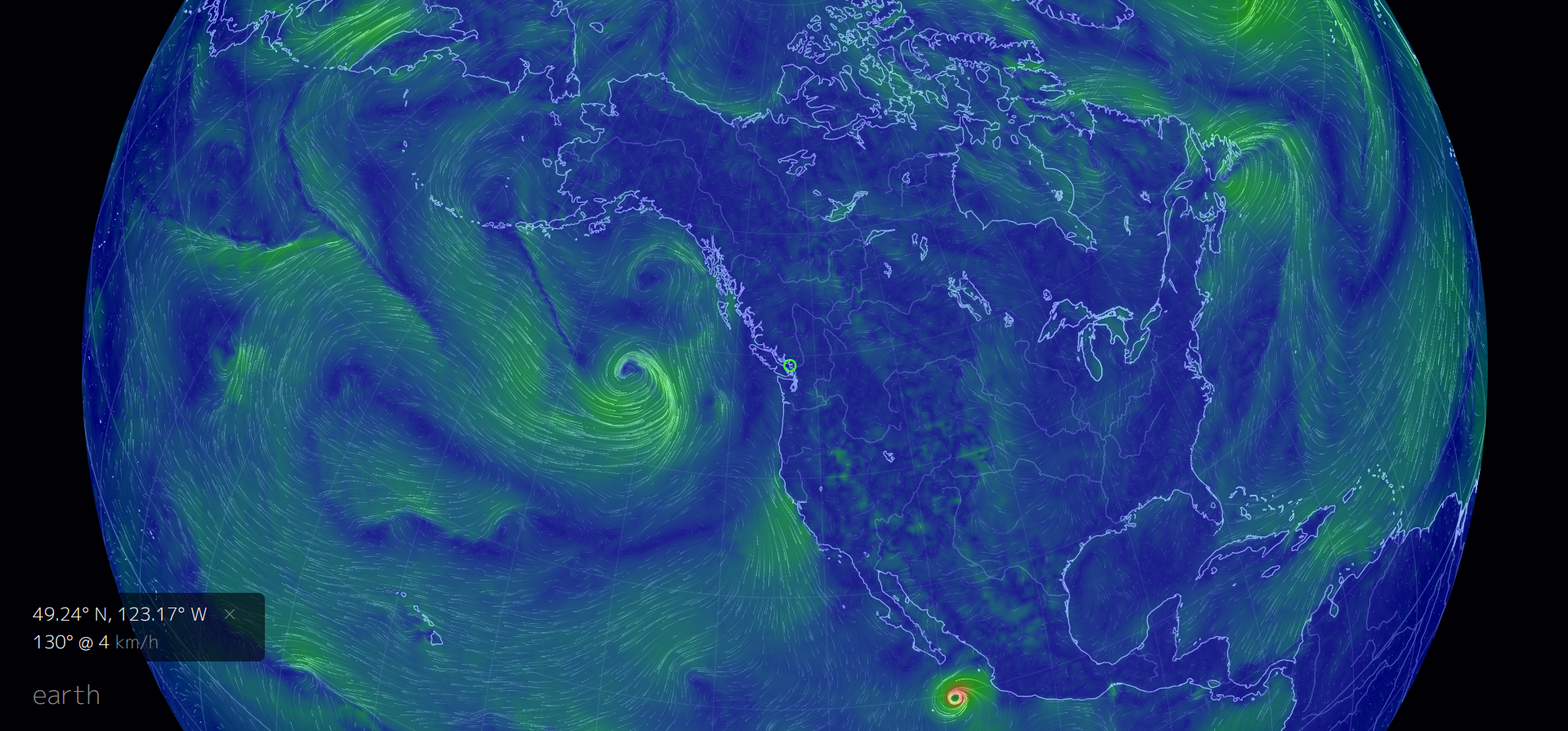# Lab 03: Atmospheric Structure and Pressure Systems

Leonard Tang

This lab is designed for you to gain an understanding of the basic structure of our atmosphere using real data. In addition, you will also learn the different types of pressure systems, how to identify them, and their relationships with atmospheric circulation (wind).

Learning Objectives

After completion of this lab, students will be able to:

• Identify the different temperature layers in the lower atmosphere;
• Produce a sounding using an Excel spreadsheet;
• Understand and calculate the environmental lapse rate;
• Identify high- and low-pressure systems; and
• Investigate the relationship between pressure systems and wind.

If you need a quick review, read Atmospheric Structure Part 1, Atmospheric Structure Part 2, and Pressure Systems and Circulation.

One of the exercises in this lab requires students to use the earth website. In their menu, they list the height of the atmosphere using hectopascals (hPa). For reference, pascal (Pa) is the SI unit of measurement for pressure. One hectopascal is equivalent to 100 pascals or 0.1 kilopascals (kPa). The average sea-level pressure is 1013.25 hPa. As such, the approximate heights of the different pressure are as follows:

Pressure (hPa) Approximate Height (km above sea-level)
1000 0
850 1.5
700 3.0
500 5.5
250 10.0
70 18.5
10 31.0

# Lab Exercises

You will need a web browser (Chrome or Firefox preferable), Excel, and a calculator to complete the lab assignment.

EX1: Construct a Sounding (Temperature Profile) of the Lower Atmosphere

In this part of the exercise, you will obtain temperature data and plot them on a graph to produce a sounding.

Step 1. Go to this location on EarthWindMap. The green circle on the map is the approximate location of Vancouver (Figure 3.1 below). At the bottom left of the map, you will see the coordinates of Vancouver, the wind direction (expressed as azimuth), wind speed, and air temperature at the surface of the location.

Step 2. Click on the label “earth” at the bottom left and the menu will expand. You have the option of choosing many different types of data (e.g. wind, temperature, relative humidity, etc.), source (e.g. GFS, NCEP, etc.), height (e.g. surface, 1000 hPa, etc.), and more. For the purpose of this exercise, we will focus only on temperature data at different heights. For example, in Figure 3.2 the temperature in Vancouver at the surface is 16.6°C, on August 18th, 2020 at 20:00 local time.

Step 3. Obtain temperature data at the different elevations starting at 1000 hPa using the “Height” option in the menu. Record the data in an Excel spreadsheet. When you’re finished, your spreadsheet should look something similar to this:

Step 4. Before you plot the data, reverse the columns so that temperature is the left column and pressure is the right column. Then select all the data including the title. Use the Insert tab in the Excel window to insert a Chart. Choose a scatter chart. A chart should appear on your spreadsheet. Hopefully, it will show a straight line with pressure values on the vertical axis and temperature values on the horizontal axis. But we want the pressure values to be the highest at the bottom, to correspond to the high pressures at the bottom of the atmosphere. To do this, double click on the numbers in the vertical axis and a Format Axis box should appear. In the Axis Options section, click on the check box for Values in reverse order near the bottom.

Step 5. Complete the graph by adding a title and labeling the axes (with units). The resulting graph shows the vertical temperature variation of the lower atmosphere, also known as a sounding.Figure 3.3. Atmospheric sounding in Vancouver, August 18, 2020 at 2000 PDT. Source: Leonard Tang. CC BY-NC-SA.

EX2: Identifying Temperature Layers of the Atmosphere

On the sounding you plotted in the previous exercise, label the locations of the troposphere, tropopause, and stratosphere. To put labels on your graph, you can add textboxes. Use arrows to show the extent of the troposphere and stratosphere.

EX3: Calculating Lapse Rate

Using the data collected, we can calculate the lapse rate of the troposphere (technically called the environmental lapse rate). To do that, first take the difference in temperature between 1000 hPa and the tropopause. For simplicity, assume the tropopause is at 12 km above sea level.  (In actuality, the height of the tropopause varies with latitude and time of year). Next, divide the difference in temperature by the height of the tropopause. For example:The lapse rate is therefore 6.33°C/km, meaning the temperature drops by about 6°C for each kilometre we go up in the troposphere.

The concept and the importance of lapse rate is examined in Lab 07 under the topic of Atmospheric Stability.

EX4: Pressure Systems and Their Relationships With Wind

Atmospheric pressure is the weight of air exerted on a surface. Wind is a direct result of the difference in atmospheric pressure between two places (i.e., the pressure gradient between locations). This means that if we know the spatial distribution of pressure systems over a certain area, we can determine the wind patterns in that same area.

For example, using the following image from the EarthWindMap website (Figure 3.4), wind patterns can be visualized. These wind patterns can help us identify where the locations of the major high- and low-pressure systems are. (Recall that winds blow outward in a clockwise direction, whereas winds blow inward in a counterclockwise direction.)

Your instructor will provide you with a map comparable to Figure 3.4. Annotate the map by adding “H” and “L” where the main pressure systems areFigure 3.4. Wind patterns in North America. Source: Leonard Tang, generated using EarthWindMap by Cameron Beccario. CC BY-NC-SA.

Another common method to look at pressure systems is to look at an actual weather map. Figure 3.5 is a surface weather map produced by Environment Canada. Notice the isobars drawn on the map, as well as the areas of high pressure (“H”) and low pressure (“L”). Draw arrows on the map (in Worksheets) to indicate the wind directions based on where the high and low pressures are located. You should draw 3-4 arrows for each pressure system on the map.

Reflection Questions

1. Using the data you collected in EX1, briefly explain why temperature varies in the troposphere. (For example, if you climb a mountain 3 km tall at the location you chose, how and why would the temperature change?)
2. If the temperature variation in the troposphere is reversed (i.e. temperature increases with elevation) what phenomenon is it?
3. In your own words, explain how pressure systems and wind are related. How and why are the spacing of isobars on a weather map important?

# Worksheets

Surface Analysis Map